# Snippet Wednesday - Spice up your progress messages with ASCII art 🎨

Communicating progress to the user during long running calculations and optimizations is important. Users often get annoyed when they only see this message for 20 minutes or more: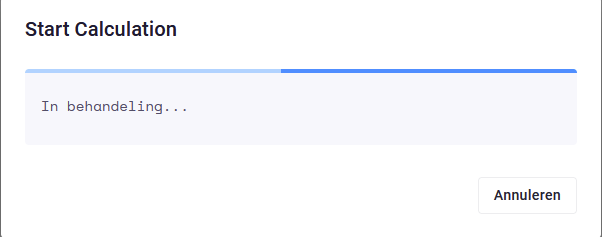Also an end user could start doubting if the calculation is still running and is not stuck or crashed.
For this purpose, progress messages were introduced. I would like to show some ways to inform the end user of the progress during calculations.

Showing progress using the total number of calculations and the percentage argument on the progress message:

``````def calculation(self, **kwargs):

# get items to be calculated
calculations_items = get_calculation_items()

# check how many calculations need to be performed and estimate the total time
total_calculations = len(calculations_items)
estimated_time = timedelta(seconds=1) * total_calculations

for i, item in enumerate(calculations_items):
# make progress message
progress_message(f"Calculation {i + 1}/{total_calculations} (estimated time: {estimated_time})",
percentage=(i / total_calculations) * 100)

some_awesome_calculation(item)

``````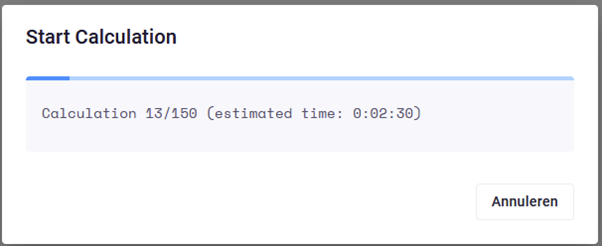Here also an estimation is given of the total time all calculations will take. This allows the end user to do something else and come back when all calculations are finished.

## Intermediate results

You could also share some intermediate results with the end user.

``````def calculation(self, **kwargs):

# get items to be calculated
calculations_items = get_calculation_items()

# check how many calculations need to be performed and estimate the total time
total_calculations = len(calculations_items)
estimated_time = timedelta(seconds=1) * total_calculations

last_result = None

for i, item in enumerate(calculations_items):

# make progress message
message = f"Calculation {i + 1}/{total_calculations} (estimated time: {estimated_time})"
if last_result:
# Concat base message with last result message
message = message + "  \n  \n" + last_result

progress_message(message,
percentage=(i / total_calculations) * 100)

result = some_awesome_calculation(item)

last_result = f"The following calculation is performed  \n" \
f"Input: some_input_parameters  \n" \
f"Result: {result}kNm"
``````

Resulting in: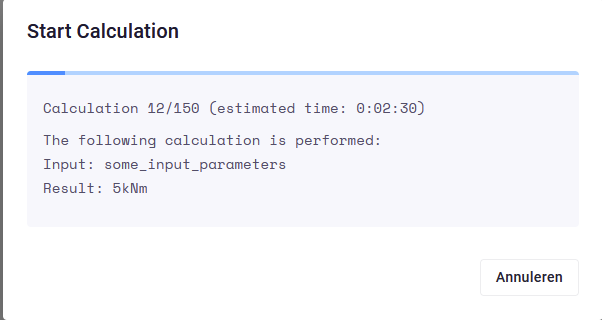## Spice it up with ASCII art

You could even consider spicing the progress message up with a little ASCII art. We can make a train move along the bottom of the message using the following code:

First, we generate the ASCII art using the following function:

``````def get_train(i):

padding = " " * (i % 45)

train = f"{padding}____  \n " \
f"{padding} |_ |_____|<  \n " \

return train

``````

This function takes the loop variable `i` to generate a padding string. The padding is added to make the train move from left to right.

Using the train, the progress message is constructed:

``````for i, item in enumerate(calculations_items):

moving_train = get_train(i)

# make progress message
message = f"Calculation {i + 1}/{total_calculations} (estimated time: {estimated_time})"
message = message + "  \n  \n" + moving_train

progress_message(message,
percentage=(i / total_calculations) * 100)

result = some_awesome_calculation(item)

``````

Result: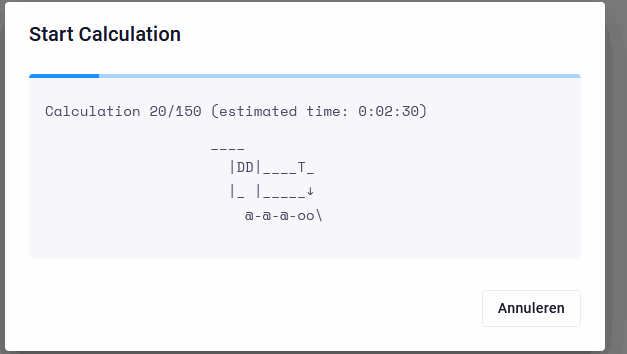I hope this inspires you to implement some awesome progress messages that keep the end user both informed and entertained. Please share your progress message design below this post!

6 Likes

Very inspiring and fun, to spice it up even more you can add some moving wheels and steam clouds``````def get_train(i):
padding = " " * (i % 45)

steam_length = i % 4
steam_padding = 12 - (steam_length * 3)

steam_top = " " * steam_padding + steam_length * "◜◝"
steam_bottom = " " * steam_padding + steam_length * "◟◞"

train = (
f"{padding}  ____   ( )  \n "
)

if i % 2 == 0:
train = train.replace("⨂", "⨁")

return train
``````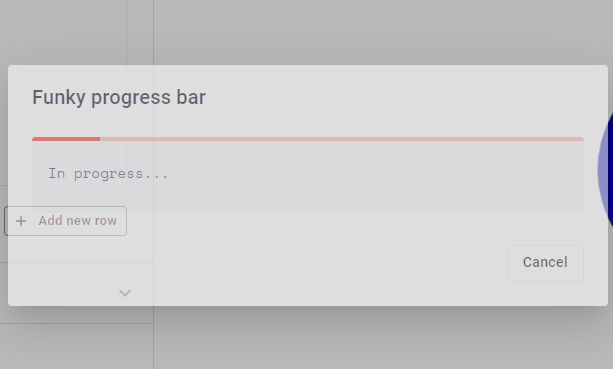6 Likes

Really cool stuff! Can’t wait to see what other developers come up with!

As the post mentions, showing progress messages is particularly useful for long-running calculations. While I think that every application should always include progress messages to inform the user and keep them engaged, to create the best possible user experience, developers should note that:

• sending a progress-message to the Web Interface is a slightly slower operation compared to other python logic in your App (it performs an API request behind the scenes)
• the (web) User Interface gets updated roughly every second to show the latest progress-message.

It therefore does not make sense to update your progress message too frequently (e.g. 50 updates per second). Not only does it not directly update the progress-message as seen in the UI, but it might actually slow down your code a bit (since updating the progress-message is a time-consuming operation).

If you for example have a calculation that does a LOT of iterations in a very short time, it might be smart to only update your progress-message every N number of iterations. Please see the example below:

``````def brute_force_analysis(input, number_iterations = 1_000):
"""Brute force analysis of Beam design

Average duration for 1,000 design exploration: 10 seconds --> 100 per second
"""
results = []
for i in range(number_iterations + 1):
if i % 100 == 0:
progress_message(f"Calculation {i}/{number_iterations}")
result = quick_analysis(input, i)
results.append(result)
...
``````
2 Likes